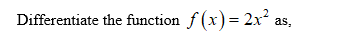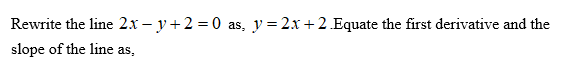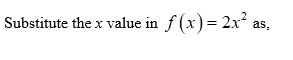# I am just stuck on this question: Find an equation of the line that is tangent to the graph of f and parallel to the given line.Function        Linef(x) = 2x2    2x − y + 2 = 0Y= _________________________ (answer)Thank you

Question
20 views

I am just stuck on this question:

Find an equation of the line that is tangent to the graph of f and parallel to the given line.

 Function Line f(x) = 2x2 2x − y + 2 = 0

Thank you

check_circle

star
star
star
star
star
1 Rating
Step 1Step 2Step 3...

### Want to see the full answer?

See Solution

#### Want to see this answer and more?

Solutions are written by subject experts who are available 24/7. Questions are typically answered within 1 hour.*

See Solution
*Response times may vary by subject and question.
Tagged in

### Other